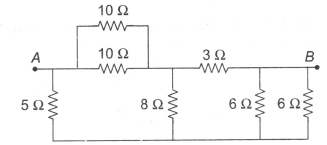Seven resistances are connected as shown in the figure. The equivalent resistance between A and B is

# Seven resistances are connected as shown in the figure. The equivalent resistance between A and B is1. A

$3\text{\hspace{0.17em}\hspace{0.17em}}\Omega$

2. B

$4\text{\hspace{0.17em}\hspace{0.17em}}\Omega$

3. C

$4.5\text{\hspace{0.17em}\hspace{0.17em}}\Omega$

4. D

$5\text{\hspace{0.17em}\hspace{0.17em}}\Omega$

Fill Out the Form for Expert Academic Guidance!l

+91

Live ClassesBooksTest SeriesSelf Learning

Verify OTP Code (required)

### Solution:

The given circuit can be simplified as follows:

Now it is a balanced Wheatstone bridge.
So,

$⇒\text{\hspace{0.17em}\hspace{0.17em}\hspace{0.17em}\hspace{0.17em}}{\mathrm{R}}_{\mathrm{AB}}\text{\hspace{0.17em}}=\text{\hspace{0.17em}}\frac{8\text{\hspace{0.17em}}×\text{\hspace{0.17em}}8}{8\text{\hspace{0.17em}}+\text{\hspace{0.17em}}8}\text{\hspace{0.17em}}=\frac{64}{16}\text{\hspace{0.17em}}=\text{\hspace{0.17em}}4\text{\hspace{0.17em}}\mathrm{\Omega }$

## Related content

 GK Questions on Global Warming What is Analog Computer? Types and How is it Different from Digital Computer? Possessive Nouns NEET Exam Centres in Arunachal Pradesh 2024 What is an Electric Circuit? How does it Work? GK Questions On Bihar: State Of India Plural Nouns NEET Exam Centres in Andhra Pradesh 2024 Maharashtra NEET UG Merit List 2023 5 Lesser Known Tips to Secure AIR 1 in NEET 2024+91

Live ClassesBooksTest SeriesSelf Learning

Verify OTP Code (required)